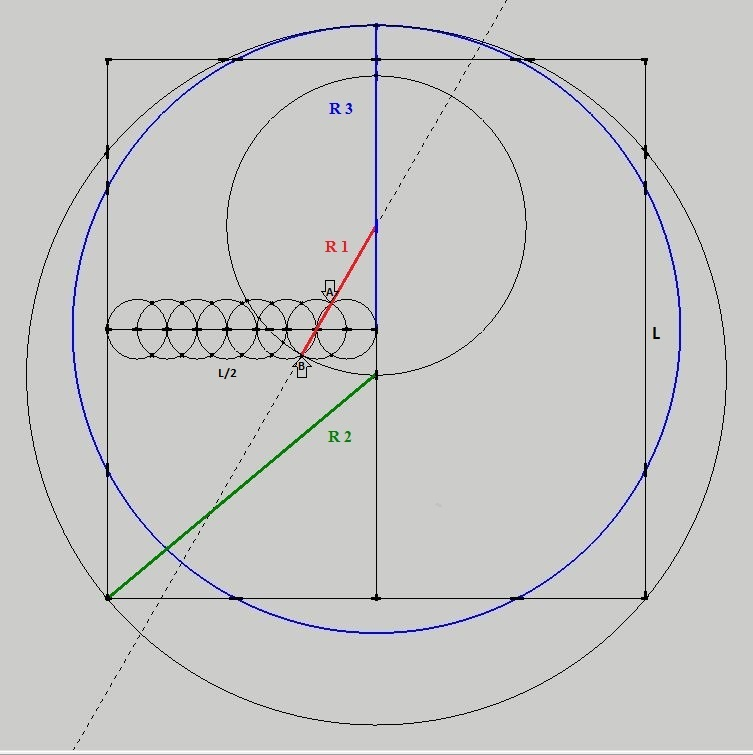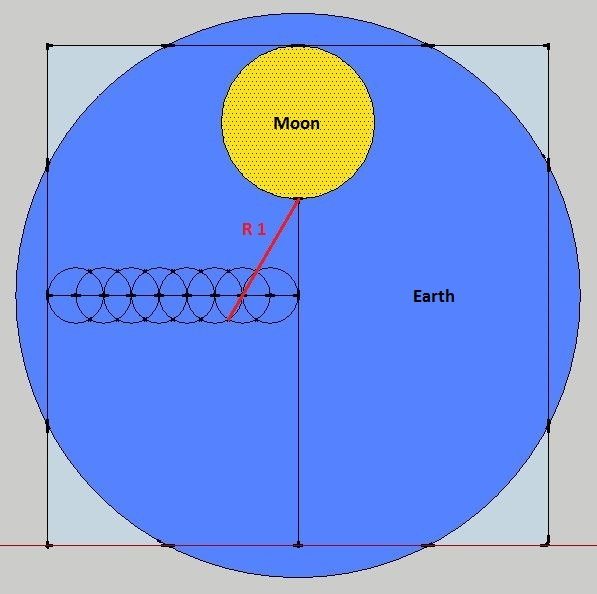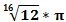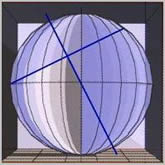Home | Study Links | Poems |

## Circling the Square

This simple, fun and elegant method to circling the square (an attempt to make a circle equal in area to that of a given square or visa versa) using a straightedge and compass only, has an accuracy that exceeds 99.9%.1. Place eight interlocking circles (L/2) along a straight line

2. Create the square (L²)3. Draw a line through points A and B and circle R1 as shown

4. Circle R2

5. Circle R3 which is the radius

><>*<><

In this circling the square method the ratio of Earth to Moon diameter is 3.66... and matches the real thing!Thank you Michael Joyce for putting this into proper mathematical format for me.

"Here follows a mathematical proof of Gertjan’s discovery.

If the radius of each of the small 8 circles is 1, then the square will have sides of length 18 (2 x 9).
D4 represents the diameter of a circle, R1, R2, R3 the radii of other circles.1- To calculate angle “a
The diagram in the lower right quadrant shows an enlargement of the second small circle
cosine a           = 0.5 divided by 1
= 1/2
Therefore a     = 60 degrees.

2- To calculate the length R1
cosine a           = 2 divided by (R1 - 1)
therefore R1    = 5

3- To calculate the length R2 using Pythagoras’ theorem:
R2²        = GH² + 9²
GH         = 18 – DG
GH         = 9 - FG

sin 60°  = EF divided by 4
EF         = 4 x sin 60°           = 3.4641016151
FG         = EG(R1) - EF          = 1.5358983848
GH        = 9 - 1.535898384    = 7.4641016151

R2²        =  GH² + 9²
= (7.4641016151)² + 81
= 136.7128129211
R2         = square root (136.7128129211)
= 11.6924254507

4- To calculate the length R3
R3         = R2 - FG
= 11.6924254507 - 1.5358983848
= 10.1565270658

Squaring the Circle.
Area of square = 18 x 18                                                           = 324
Area of circle   = pi x R3² = 3.141… x 10.1565270658 ²           = 324.071122253... (Q.E.D)

5- To calculate the length D4
D4         = DF - 4 x sin 60°
= 9 - 3.4641016151
= 5.5358983848

Ratio of (2 x R3 = diameter of circle) : D4 compared with Earth : Moon diameters
(10.1565270658 x 2) : 5.5358983848 = 20.3130541317 : 5.5358983848
Or 3.66933 : 1

The ratio of diameters Earth : Moon as 7920 : 2160 (11:3)
Or 3.66666 : 1

Answers are the same with negligible error ‼"

Since the Earth and Moon are only approximately spherical; no single value can serve as a definitive radius.
For example, the Earth’s meaningful values range from 6355 to 6378 kilometres (3949 to 3963 mi).
However, the Earth and Moon will be exact spheres in an absolute reality.
Perhaps with ratio of diameters= 3.66943... : 1